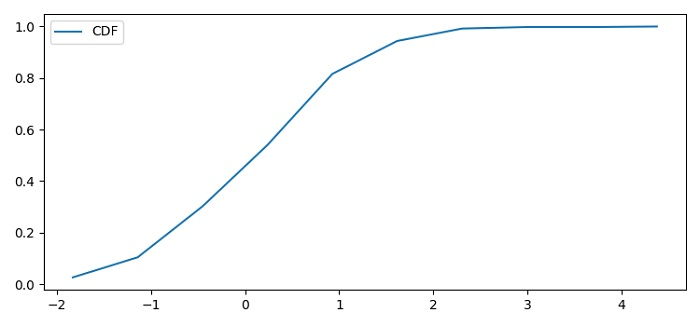# How to plot cdf in Matplotlib in Python?

To plot cdf in matplotlib in Python, we can take the following steps −

• Set the figure size and adjust the padding between and around the subplots.

• Initialize a variable N for the number of sample data.

• Create random data using numpy.

• Compute the histogram of a set of data with data and bins=10.

• Find the probability distribution function (pdf).

• Using pdf (Step 5), calculate cdf.

• Plot the cdf using plot() method with label "CDF".

• Place a legend on the plot.

• To display the figure, use show() method.

## Example

import numpy as np
from matplotlib import pyplot as plt

plt.rcParams["figure.figsize"] = [7.50, 3.50]
plt.rcParams["figure.autolayout"] = True

N = 500
data = np.random.randn(N)
count, bins_count = np.histogram(data, bins=10)
pdf = count / sum(count)
cdf = np.cumsum(pdf)
plt.plot(bins_count[1:], cdf, label="CDF")
plt.legend()
plt.show()

## Output